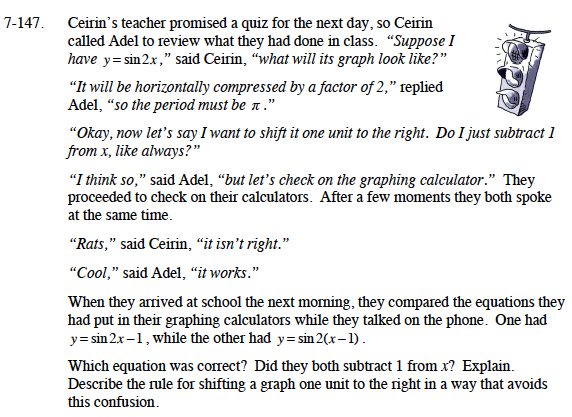Home > CCA2 > Chapter 7 > Lesson 7.2.3 > Problem7-147

7-147.
1.Ceirin’s teacher promised a quiz for the next day, so Ceirin called Adel to review what they had done in class. “Suppose I have y = sin2x,” said Ceirin, “what will its graph look like?”

2. “It will be horizontally compressed by a factor of 2,”replied Adel, “so the period must be π.”

3. “Okay, now let’s say I want to shift it one unit to the right. Do I just subtract 1 from x, like always?”

4. “I think so,” said Adel, “but let’s check on the graphing calculator.” They proceeded to check on their calculators. After a few moments they both spoke at the same time.

5. Rats,” said Ceirin, “it isn’t right.”

6. “Cool,” said Adel, “it works.”

7. When they arrived at school the next morning, they compared the equations they had put in their graphing calculators while they talked on the phone. One had y = sin 2x − 1, while the other had y = sin2(x − 1).

8. Which equation was correct? Did they both subtract 1 from x? Explain. Describe the rule for shifting a graph one unit to the right in a way that avoids this confusion. Homework Help ✎If you want to subtract 1 from x before multiplying, are parenthesis necessary?

The equation y = sin2(x − 1) is correct. To shift the graph one unit to the right, subtract 1 from x before multiplying by anything.